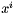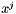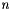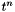# Subpolynomials

## Functions

void fmpq_poly_getslice (fmpq_poly_ptr rop, const fmpq_poly_ptr op, ulong i, ulong j)
Returns a contiguous subpolynomial.Returns the slice with coefficients from(including) to(excluding).
void fmpq_poly_left_shift (fmpq_poly_ptr rop, const fmpq_poly_ptr op, ulong n)
Shifts this polynomial to the left bycoefficients.Notionally multiplies `op` byand stores the result in `rop`.
void fmpq_poly_right_shift (fmpq_poly_ptr rop, const fmpq_poly_ptr op, ulong n)
Shifts this polynomial to the right bycoefficients.Notationally returns the quotient of floor division of `rop` by `op`.
void fmpq_poly_truncate (fmpq_poly_ptr rop, const fmpq_poly_ptr op, ulong n)
Truncates this polynomials.Truncates `op` modulowheneveris positive. Returns zero otherwise.
void fmpq_poly_reverse (fmpq_poly_ptr rop, const fmpq_poly_ptr op, ulong n)
Reverses this polynomial.Reverses the coefficients of `op` - thought of as a polynomial of length `n` - and places the result in `rop`.

## Function Documentation

 void fmpq_poly_getslice ( fmpq_poly_ptr rop, const fmpq_poly_ptr op, ulong i, ulong j )

Returns a contiguous subpolynomial.Returns the slice with coefficients from(including) to(excluding).

 void fmpq_poly_left_shift ( fmpq_poly_ptr rop, const fmpq_poly_ptr op, ulong n )

Shifts this polynomial to the left bycoefficients.Notionally multiplies `op` byand stores the result in `rop`.

 void fmpq_poly_reverse ( fmpq_poly_ptr rop, const fmpq_poly_ptr op, ulong n )

Reverses this polynomial.Reverses the coefficients of `op` - thought of as a polynomial of length `n` - and places the result in `rop`.

 void fmpq_poly_right_shift ( fmpq_poly_ptr rop, const fmpq_poly_ptr op, ulong n )

Shifts this polynomial to the right bycoefficients.Notationally returns the quotient of floor division of `rop` by `op`.

 void fmpq_poly_truncate ( fmpq_poly_ptr rop, const fmpq_poly_ptr op, ulong n )

Truncates this polynomials.Truncates `op` modulowheneveris positive. Returns zero otherwise.

Generated on Wed Dec 8 21:13:35 2010 for FMPQ_POLY by1.6.3